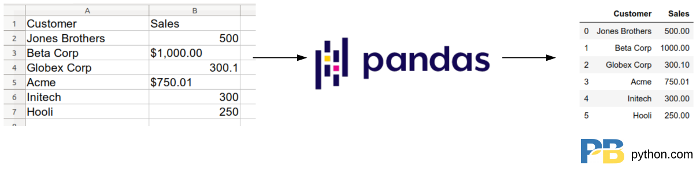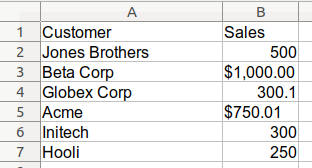Taking care of business, one python script at a time

Mon 28 October 2019

# Cleaning Up Currency Data with Pandas

Posted by Chris Moffitt in articles## Introduction

The other day, I was using pandas to clean some messy Excel data that included several thousand rows of inconsistently formatted currency values. When I tried to clean it up, I realized that it was a little more complicated than I first thought. Coincidentally, a couple of days later, I followed a twitter thread which shed some light on the issue I was experiencing. This article summarizes my experience and describes how to clean up messy currency fields and convert them into a numeric value for further analysis. The concepts illustrated here can also apply to other types of pandas data cleanup tasks.

## The Data

Here is a simple view of the messy Excel data:In this example, the data is a mixture of currency labeled and non-currency labeled values. For a small example like this, you might want to clean it up at the source file. However, when you have a large data set (with manually entered data), you will have no choice but to start with the messy data and clean it in pandas.

Before going further, it may be helpful to review my prior article on data types. In fact, working on this article drove me to modify my original article to clarify the types of data stored in ``` object``` columns.

```import pandas as pd

df = df_orig.copy()
```
Customer Sales
0 Jones Brothers 500
1 Beta Corp \$1,000.00
2 Globex Corp 300.1
3 Acme \$750.01
4 Initech 300
5 Hooli 250

I’ve read in the data and made a copy of it in order to preserve the original.

One of the first things I do when loading data is to check the types:

```df.dtypes
```
```Customer    object
Sales       object
dtype: object
```

Not surprisingly the ``` Sales``` column is stored as an object. The ‘\$’ and ‘,’ are dead giveaways that the ``` Sales``` column is not a numeric column. More than likely we want to do some math on the column so let’s try to convert it to a float.

In the real world data set, you may not be so quick to see that there are non-numeric values in the column. In my data set, my first approach was to try to use ``` astype()```

```df['Sales'].astype('float')
```
```---------------------------------------------------------------------------
ValueError                                Traceback (most recent call last)
<ipython-input-50-547a9c970d4a> in <module>
----> 1 df['Sales'].astype('float')

.....
ValueError: could not convert string to float: '\$1,000.00'
```

The traceback includes a ``` ValueError``` and shows that it could not convert the \$1,000.00 string to a float. Ok. That should be easy to clean up.

Let’s try removing the ‘\$’ and ‘,’ using ``` str.replace``` :

```df['Sales'] = df['Sales'].str.replace(',', '')
df['Sales'] = df['Sales'].str.replace('\$', '')
df['Sales']
```
```0        NaN
1    1000.00
2        NaN
3     750.01
4        NaN
5        NaN
Name: Sales, dtype: object
```

Hmm. That was not what I expected. For some reason, the string values were cleaned up but the other values were turned into ``` NaN``` . That’s a big problem.

To be honest, this is exactly what happened to me and I spent way more time than I should have trying to figure out what was going wrong. I eventually figured it out and will walk through the issue here so you can learn from my struggles!

The twitter thread from Ted Petrou and comment from Matt Harrison summarized my issue and identified some useful pandas snippets that I will describe below.

Basically, I assumed that an ``` object``` column contained all strings. In reality, an object column can contain a mixture of multiple types.

Let’s look at the types in this data set.

```df = df_orig.copy()
df['Sales'].apply(type)
```
```0      <class 'int'>
1      <class 'str'>
2      <class 'float'>
3      <class 'str'>
4      <class 'int'>
5      <class 'int'>
Name: Sales, dtype: object
```

Ahhh. This nicely shows the issue. The ``` apply(type)``` code runs the ``` type``` function on each value in the column. As you can see, some of the values are floats, some are integers and some are strings. Overall, the column ``` dtype``` is an object.

Here are two helpful tips, I’m adding to my toolbox (thanks to Ted and Matt) to spot these issues earlier in my analysis process.

First, we can add a formatted column that shows each type:

```df['Sales_Type'] = df['Sales'].apply(lambda x: type(x).__name__)
```
Customer Sales Sales_Type
0 Jones Brothers 500 int
1 Beta Corp \$1,000.00 str
2 Globex Corp 300.1 float
3 Acme \$750.01 str
4 Initech 300 int
5 Hooli 250 int

Or, here is a more compact way to check the types of data in a column using ``` value_counts()``` :

```df['Sales'].apply(type).value_counts()
```
```<class 'int'>      3
<class 'str'>      2
<class 'float'>    1
Name: Sales, dtype: int64
```

I will definitely be using this in my day to day analysis when dealing with mixed data types.

## Fixing the Problem

To illustrate the problem, and build the solution; I will show a quick example of a similar problem using only python data types.

First, build a numeric and string variable.

```number = 1235
number_string = '\$1,235'
print(type(number_string), type(number))
```
```<class 'str'> <class 'int'>
```

This example is similar to our data in that we have a string and an integer. If we want to clean up the string to remove the extra characters and convert to a float:

```float(number_string.replace(',', '').replace('\$', ''))
```
```1235.0
```

Ok. That’s what we want.

What happens if we try the same thing to our integer?

```float(number.replace(',', '').replace('\$', ''))
```
```---------------------------------------------------------------------------
AttributeError                            Traceback (most recent call last)
<ipython-input-66-fe0f3ed32c3e> in <module>
----> 1 float(number.replace(',', '').replace('\$', ''))

AttributeError: 'int' object has no attribute 'replace'
```

There’s the problem. We get an error trying to use string functions on an integer.

When pandas tries to do a similar approach by using the ``` str``` accessor, it returns an ``` NaN``` instead of an error. That’s why the numeric values get converted to ``` NaN``` .

The solution is to check if the value is a string, then try to clean it up. Otherwise, avoid calling string functions on a number.

The first approach is to write a custom function and use ``` apply``` .

```def clean_currency(x):
""" If the value is a string, then remove currency symbol and delimiters
otherwise, the value is numeric and can be converted
"""
if isinstance(x, str):
return(x.replace('\$', '').replace(',', ''))
return(x)
```

This function will check if the supplied value is a string and if it is, will remove all the characters we don’t need. If it is not a string, then it will return the original value.

Here is how we call it and convert the results to a float. I also show the column with the types:

```df['Sales'] = df['Sales'].apply(clean_currency).astype('float')
df['Sales_Type'] = df['Sales'].apply(lambda x: type(x).__name__)
```
Customer Sales Sales_Type
0 Jones Brothers 500.00 float
1 Beta Corp 1000.00 float
2 Globex Corp 300.10 float
3 Acme 750.01 float
4 Initech 300.00 float
5 Hooli 250.00 float

We can also check the ``` dtypes``` :

```df.dtypes
```
```Customer       object
Sales         float64
Sales_Type     object
dtype: object
```

Or look at the ``` value_counts``` :

```df['Sales'].apply(type).value_counts()
```
```<class 'float'>    6
Name: Sales, dtype: int64
```

Ok. That all looks good. We can proceed with any mathematical functions we need to apply on the sales column.

Before finishing up, I’ll show a final example of how this can be accomplished using a lambda function:

```df = df_orig.copy()
df['Sales'] = df['Sales'].apply(lambda x: x.replace('\$', '').replace(',', '')
if isinstance(x, str) else x).astype(float)
```

The lambda function is a more compact way to clean and convert the value but might be more difficult for new users to understand. I personally like a custom function in this instance. Especially if you have to clean up multiple columns.

The final caveat I have is that you still need to understand your data before doing this cleanup. I am assuming that all of the sales values are in dollars. That may or may not be a valid assumption.

If there are mixed currency values here, then you will need to develop a more complex cleaning approach to convert to a consistent numeric format. Pyjanitor has a function that can do currency conversions and might be a useful solution for more complex problems.

## Alternative Solutions

After I originally published the article, I received several thoughtful suggestions for alternative ways to solve the problem. The first suggestion was to use a regular expression to remove the non-numeric characters from the string.

```df['Sales'] = df['Sales'].replace({'\\$': '', ',': ''}, regex=True).astype(float)
```

This approach uses pandas Series.replace. It looks very similar to the string replace approach but this code actually handles the non-string values appropriately.

Regular expressions can be challenging to understand sometimes. However, this one is simple so I would not hesitate to use this in a real world application. Thanks to Serg for pointing this out.

The other alternative pointed out by both Iain Dinwoodie and Serg is to convert the column to a string and safely use ``` str.replace.```

First we read in the data and use the ``` dtype``` argument to ``` read_excel``` to force the original column of data to be stored as a string:

```df = pd.read_excel('sales_cleanup.xlsx', dtype={'Sales': str})
```

We can do a quick check:

```df['Sales'].apply(type).value_counts()
```
```<class 'str'>    6
Name: Sales, dtype: int64
```

Then apply our cleanup and type conversion:

```df['Sales'] = df['Sales'].str.replace(',','').str.replace('\$','').astype('float')
```

Since all values are stored as strings, the replacement code works as expected and does not incorrectly convert some values to ``` NaN.```

## Summary

The pandas ``` object``` data type is commonly used to store strings. However, you can not assume that the data types in a column of pandas ``` objects``` will all be strings. This can be especially confusing when loading messy currency data that might include numeric values with symbols as well as integers and floats.

It is quite possible that naive cleaning approaches will inadvertently convert numeric values to ``` NaN``` . This article shows how to use a couple of pandas tricks to identify the individual types in an object column, clean them and convert them to the appropriate numeric value.

I hope you have found this useful. If you have any other tips or questions, let me know in the comments.

## Changes

• 3-Nov-2019: Updated article to include a link to the data and highlight some alternative solutions provided in the comments.